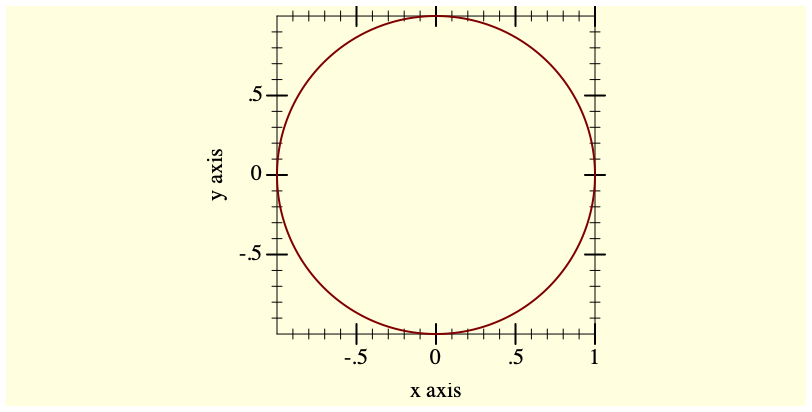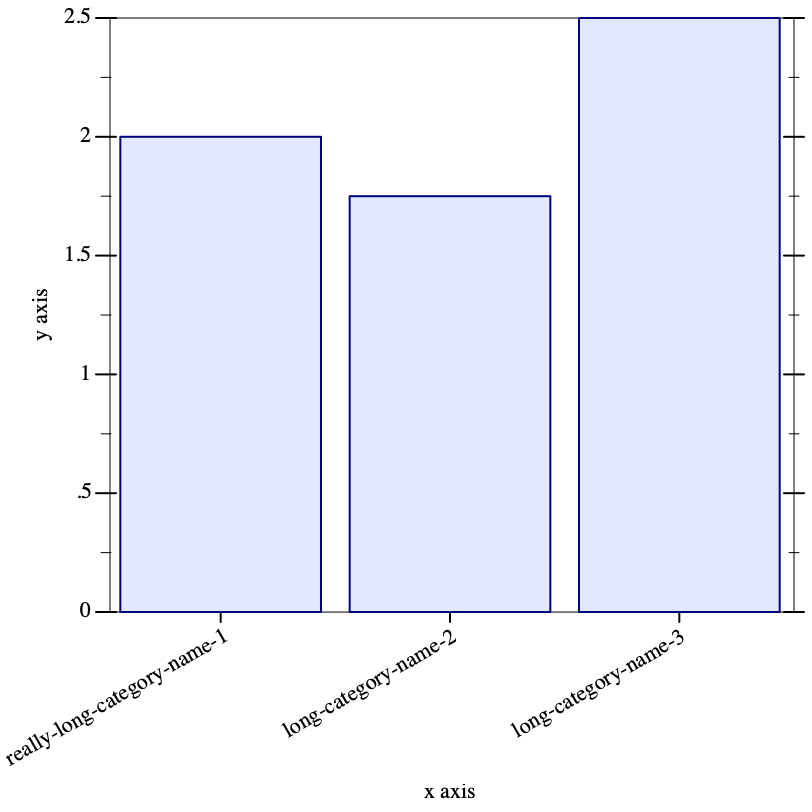8.4.0.3

9Plot Contracts

 (require plot/utils) package: plot-lib

9.1Plot Element Contracts

 procedure(renderer2d? value) → boolean? value : any/c
Returns #t if value is a 2D renderer; that is, if plot can plot value. See 2D Renderers for functions that construct them.

 procedure(renderer3d? value) → boolean? value : any/c
Returns #t if value is a 3D renderer; that is, if plot3d can plot value. See 3D Renderers for functions that construct them.

 procedure(nonrenderer? value) → boolean? value : any/c
Returns #t if value is a nonrenderer. See Nonrenderers for functions that construct them.

 procedure(treeof elem-contract) → contract? elem-contract : contract?
Identifies values that meet the contract elem-contract, lists of such values, lists of lists, and so on.

9.2Appearance Argument Contracts

value

=
 (one-of/c 'top-left    'top    'top-right 'left        'center 'right 'bottom-left 'bottom 'bottom-right 'auto)
The contract for anchor arguments and parameters.

The 'auto anchor will place labels so they are visible on the plot area. This anchor type is useful for point-label and similar renderers where the labeled point might be at the edge of the plot area and the user does not wish to calculate the exact anchor for the label.

The 'auto anchor will choose one of the 'bottom-left, 'bottom-right, 'top-left or 'top-right placements, in that order, and will use the first one that would result in the label being completely visible.

The 'auto anchor is only valid for placement of text labels, for all other use cases, the 'auto anchor is always the same as 'bottom-left.

value

=
 (or/c anchor/c (one-of/c 'outside-top-left    'outside-top    'outside-top-right 'outside-left-top    'outside-left   'outside-left-bottom 'outside-right-top   'outside-right  'outside-right-bottom 'outside-bottom-left 'outside-bottom 'outside-bottom-right 'outside-global-top 'no-legend))
The contract for the plot-legend-anchor parameter and the #:legend-anchor parameters for the various plot procedures.

When legend-anchor is one of the symbols from anchor/c, the legend will be placed inside the plot area.

legend-anchor/c values which start with "outside" will place the legend outside the plot area, for 2D plots the legend will be aligned with the plot area, while for 3D plots the legend will be relative to the overall plot-width and plot-height.

The 'outside-global-top value will place the legend above the plot-area, centered on the complete plot-width. For 3D plots there is no difference between this value and 'outside-top.

The value 'no-legend, will omit the legend from the plot, the legend will also be omitted if none of the renderers have a #:label specified, regardless of the value used for #:legend-anchor.

The value 'auto, will place the legend in the top-left corner of the plot area, this is not usefull for plot legends, this anchor value is used for renderers such as point-label.

Added in version 7.9 of package plot-lib.

value

=
 (or/c (list/c real? real? real?) string? symbol? (is-a?/c color%))
A contract for very flexible color arguments. Functions that accept a color/c almost always convert it to an RGB triplet using ->color.

 value
The contract for #:color arguments, and parameters such as line-color and surface-color. For the meaning of integer colors, see ->pen-color and ->brush-color.

value

=
 (or/c exact-integer? (one-of/c 'transparent 'solid    'dot 'long-dash 'short-dash  'dot-dash))
The contract for #:style arguments when they refer to lines, and paramters such as line-style. For the meaning of integer pen styles, see ->pen-style.

value

=
 (or/c exact-integer? (one-of/c 'transparent      'solid 'bdiagonal-hatch  'fdiagonal-hatch 'crossdiag-hatch 'horizontal-hatch 'vertical-hatch  'cross-hatch))
The contract for #:style arguments when they refer to fills, and parameters such as interval-style. For the meaning of integer brush styles, see ->brush-style.

 value
Identifies legal font family values. The same as font-family/c from racket/draw.

 value = (or/c char? string? integer? (apply one-of/c known-point-symbols))
The contract for the #:sym arguments in points and points3d, and the parameter point-sym.

value

=
 (list 'dot               'point            'pixel 'plus              'times            'asterisk '5asterisk         'odot             'oplus 'otimes            'oasterisk        'o5asterisk 'circle            'square           'diamond 'triangle          'fullcircle       'fullsquare 'fulldiamond       'fulltriangle     'triangleup 'triangledown      'triangleleft     'triangleright 'fulltriangleup    'fulltriangledown 'fulltriangleleft 'fulltriangleright 'rightarrow       'leftarrow 'uparrow           'downarrow        '4star '5star             '6star            '7star '8star             'full4star        'full5star 'full6star         'full7star        'full8star 'circle1           'circle2          'circle3 'circle4           'circle5          'circle6 'circle7           'circle8          'bullet 'fullcircle1       'fullcircle2      'fullcircle3 'fullcircle4       'fullcircle5      'fullcircle6 'fullcircle7       'fullcircle8      'none)
A list containing the symbols that are valid points symbols.

 value = (or/c 'auto 'png 'jpeg 'xmb 'xpm 'bmp 'ps 'pdf 'svg)
A contract for an argument that describes an image file format.

9.3Appearance Argument List Contracts

procedure

(maybe-function/c in-contract out-contract)  contract?

in-contract : contract?
out-contract : contract?
 = (or/c out-contract (in-contract . -> . out-contract))
Returns a contract that accepts either a function from in-contract to out-contract, or a plain out-contract value.

> (require racket/contract)
 > (define/contract (maybe-function-of-real-consumer x) ((maybe-function/c real? real?) . -> . real?) (maybe-apply x 10))
> (maybe-function-of-real-consumer 4)

4

> (maybe-function-of-real-consumer (λ (x) x))

10

Many plot functions, such as contours and isosurfaces3d, optionally take lists of appearance values (such as (listof plot-color/c)) as arguments. A very flexible argument contract would accept functions that produce lists of appearance values. For example, contours would accept any f with contract (-> (listof real?) (listof plot-color/c)) for its #:colors argument. When rendering a contour plot, contours would apply f to a list of the contour z values to get the contour colors.

However, most uses do not need this flexibility. Therefore, plot’s functions accept either a list of appearance values or a function from a list of appropriate values to a list of appearance values. The maybe-function/c function constructs contracts for such arguments.

In plot functions, if in-contract is a listof contract, the output list’s length need not be the same as the input list’s length. If it is shorter, the appearance values will cycle; if longer, the tail will not be used.

 procedure(maybe-apply f arg) → any/c f : (maybe-function/c any/c any/c) arg : any/c
If f is a function, applies f to args; otherwise returns f.

This is used inside many renderer-producing plot functions to convert maybe-function/c values to lists of appearance values.

procedure

(plot-colors/c in-contract)  contract?

in-contract : contract?
 = (maybe-function/c in-contract (listof plot-color/c))
Returns a contract for #:colors arguments, as in contours and contour-intervals. See maybe-function/c for a discussion of the returned contract.

The following example sends a list-valued (plot-colors/c ivl?) to contour-intervals, which then cycles through the colors:
 > (plot (contour-intervals (λ (x y) (+ x y)) 0 1 0 1 #:colors '(1 2)))This is equivalent to sending (λ _ '(1 2)).

The next example is more sophisticated: it sends a function-valued (plot-colors/c ivl?) to contour-intervals. The function constructs colors from the values of the contour intervals.
 > (define (brown-interval-colors ivls) (define z-size (- (ivl-max (last ivls)) (ivl-min (first ivls)))) (for/list ([i  (in-list ivls)]) (match-define (ivl z-min z-max) i) (define z-mid (/ (* 1/2 (+ z-min z-max)) z-size)) (list (* 255 z-mid) (* 128 z-mid) (* 64 z-mid))))
 > (plot (contour-intervals (λ (x y) (+ x y)) 0 1 0 1 #:colors brown-interval-colors))procedure

(pen-widths/c in-contract)  contract?

in-contract : contract?
 = (maybe-function/c in-contract (listof (>=/c 0)))
Like plot-colors/c, but for line widths.

procedure

(plot-pen-styles/c in-contract)  contract?

in-contract : contract?
 = (maybe-function/c in-contract (listof plot-pen-style/c))
Like plot-colors/c, but for line styles.

procedure

(plot-brush-styles/c in-contract)  contract?

in-contract : contract?
 = (maybe-function/c in-contract (listof plot-brush-style/c))
Like plot-colors/c, but for fill styles.

procedure

(alphas/c in-contract)  contract?

in-contract : contract?
 = (maybe-function/c in-contract (listof (real-in 0 1)))
Like plot-colors/c, but for opacities.

procedure

(labels/c in-contract)  contract?

in-contract : contract?
 = (maybe-function/c in-contract (listof (or/c string? pict? #f)))
Like plot-colors/c, but for strings. This is used, for example, to label stacked-histograms.